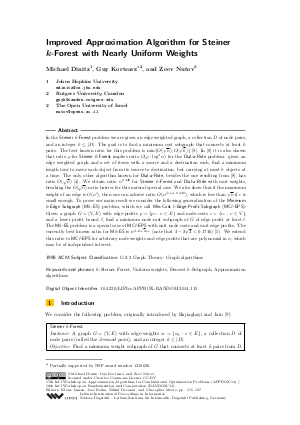Document# Improved Approximation Algorithm for Steiner k-Forest with Nearly Uniform Weights

### Authors Michael Dinitz, Guy Kortsarz, Zeev Nutov## File

LIPIcs.APPROX-RANDOM.2014.115.pdf
• Filesize: 497 kB
• 13 pages

## Cite As

Michael Dinitz, Guy Kortsarz, and Zeev Nutov. Improved Approximation Algorithm for Steiner k-Forest with Nearly Uniform Weights. In Approximation, Randomization, and Combinatorial Optimization. Algorithms and Techniques (APPROX/RANDOM 2014). Leibniz International Proceedings in Informatics (LIPIcs), Volume 28, pp. 115-127, Schloss Dagstuhl - Leibniz-Zentrum für Informatik (2014)
https://doi.org/10.4230/LIPIcs.APPROX-RANDOM.2014.115

## Abstract

In the Steiner k-Forest problem we are given an edge weighted graph, a collection D of node pairs, and an integer k \leq |D|. The goal is to find a minimum cost subgraph that connects at least k pairs. The best known ratio for this problem is min{O(sqrt{n}),O(sqrt{k})} [Gupta et al., 2008]. In [Gupta et al., 2008] it is also shown that ratio rho for Steiner k-Forest implies ratio O(rho log^2 n) for the Dial-a-Ride problem: given an edge weighted graph and a set of items with a source and a destination each, find a minimum length tour to move each object from its source to destination, but carrying at most k objects at a time. The only other algorithm known for Dial-a-Ride, besides the one resulting from [Gupta et al., 2008], has ratio O(sqrt{n}) [Charikar and Raghavachari, 1998]. We obtain ratio n^{0.448} for Steiner k-Forest and Dial-a-Ride with unit weights, breaking the O(sqrt{n}) ratio barrier for this natural special case. We also show that if the maximum weight of an edge is O(n^{epsilon}), then one can achieve ratio O(n^{(1+epsilon) 0.448}), which is less than sqrt{n} if epsilon is small enough. To prove our main result we consider the following generalization of the Minimum k-Edge Subgraph (Mk-ES) problem, which we call Min-Cost l-Edge-Profit Subgraph (MCl-EPS): Given a graph G=(V,E) with edge-profits p={p_e: e in E} and node-costs c={c_v: v in V}, and a lower profit bound l, find a minimum node-cost subgraph of G of edge profit at least l. The Mk-ES problem is a special case of MCl-EPS with unit node costs and unit edge profits. The currently best known ratio for Mk-ES is n^{3-2*sqrt{2} + epsilon} (note that 3-2*sqrt{2} < 0.1716). We extend this ratio to MCl-EPS for arbitrary node weights and edge profits that are polynomial in n, which may be of independent interest.
##### Keywords
• k-Steiner Forest; Uniform weights; Densest k-Subgraph; Approximation algorithms

## Metrics

• Access Statistics
• Total Accesses (updated on a weekly basis)
0